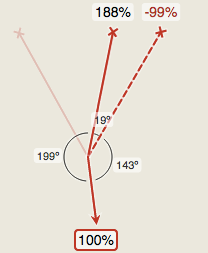# RigRite

## Calculate complex vector forces easily

• Multi-point anchors
• Redirects/Directionals
• Tripods and other AHDs
• Highlines and offsets
• Slope lower/raise
• Tension and compression
• Any units: kN, %, kg, custom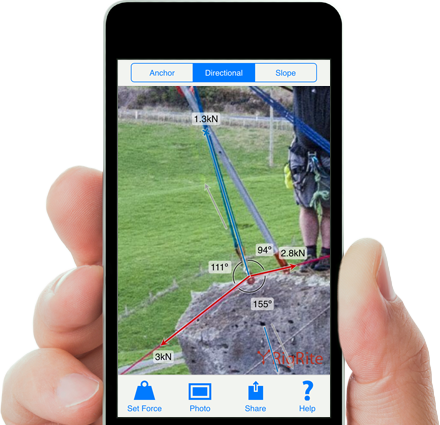## Why can't a three point anchor be calculated?

RigRite works by using vector mathematics to analyze how angles affect forces in a system. There are some things that simply cannot be worked out from angles alone, three (or more) point anchor systems is one of them.

Take the following example. It’s obvious that the anchor loading is very different in these two setups; in the first the load is suspended from the outside two anchors while in the second it’s hanging straight off the centre one. You can see that knowing the angles involved is not enough to calculate the forces. Without changing the angles drastically different loading is possible.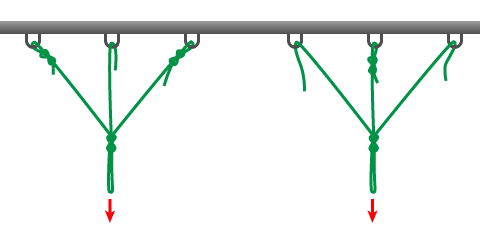This is obviously an extreme example. In most real world examples we can assume the anchor load is roughly equal. Unfortunately ‘assume roughly equal’ is not something we can put a number on. We just don’t know how close to this extreme they really are without measuring.

### What we can do

There are some things we can tell from the angles. We can calculate the range of possible forces each anchor will experience.

﻿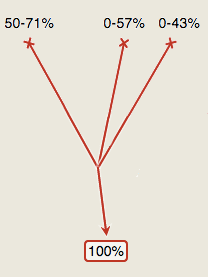We do this by assuming there is no force on a particular anchor to see the force the other two would experience in this uneven loading situation.

﻿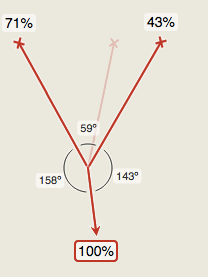﻿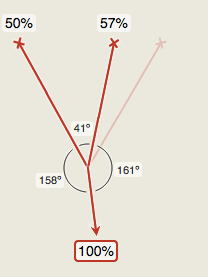There’s no need to test with just the second and third anchor because this would require the third leg to be under compression, which isn’t possible with rope.